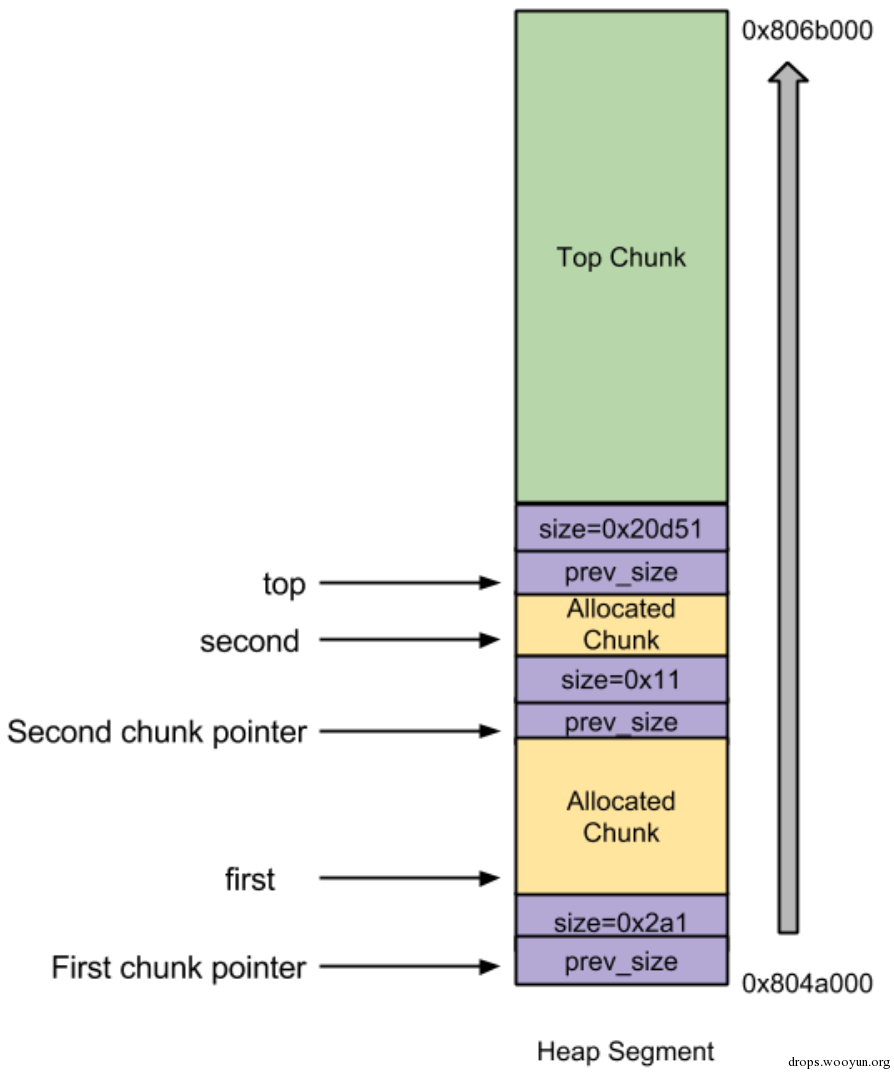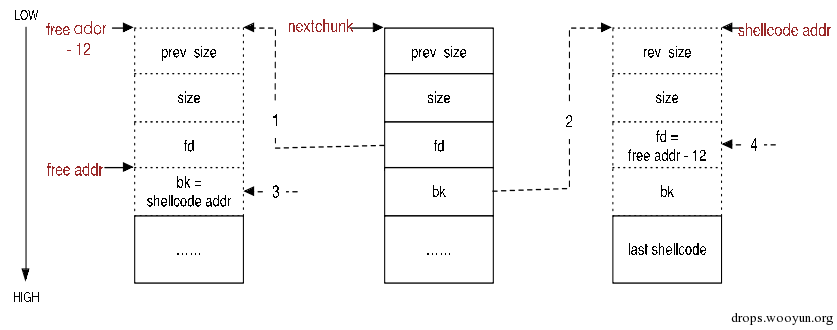# 0x01 背景介绍

``````#!c
/*
Heap overflow vulnerable program.
*/
#include <stdlib.h>
#include <string.h>

int main( int argc, char * argv[] )
{
char * first, * second;

/**/ first = malloc( 666 );
/**/ second = malloc( 12 );
if(argc!=1)
/**/         strcpy( first, argv );
/**/ free( first );
/**/ free( second );
/**/ return( 0 );
}
``````## 2.1 基本知识介绍

PS: 鉴于篇幅，这里主要介绍非mmaped的chunks的回收机制，回想一下在哪些情况下使用mmap分配新的chunk，哪些情况下不用mmap？

``````#!c
/*malloc.c  int_free函数中*/
/*这里p指向当前malloc_chunk结构体，bck和fwd分别为当前chunk的向后和向前一个free chunk*/
/* consolidate backward */
if (!prev_inuse(p)) {
prevsize = p->prev_size;
size += prevsize;
//修改指向当前chunk的指针，指向前一个chunk。
p = chunk_at_offset(p, -((long) prevsize));
}

//相关函数说明：
/* Treat space at ptr + offset as a chunk */
#define chunk_at_offset(p, s)  ((mchunkptr) (((char *) (p)) + (s)))

#define unlink(P, BK, FD) {                                            \
FD = P->fd;                                   \
BK = P->bk;                                   \
FD->bk = BK;                                  \
BK->fd = FD;                                  \
...
}
``````

1)将前一个chunk占用的内存合并到当前chunk;
2)修改指向当前chunk的指针，改为指向前一个chunk。

``````#!c
……
/*这里p指向当前chunk*/
nextchunk = chunk_at_offset(p, size);
……
nextsize = chunksize(nextchunk);
……
if (nextchunk != av->top) {
/* get and clear inuse bit */
nextinuse = inuse_bit_at_offset(nextchunk, nextsize);//判断nextchunk是否为free chunk
/* consolidate forward */
if (!nextinuse) { //next chunk为free chunk
size += nextsize; // p还是指向当前chunk只是当前chunk的size扩大了，这就是向前合并！
} else
clear_inuse_bit_at_offset(nextchunk, 0);

……
}
``````

``````#!c
/*
Place the chunk in unsorted chunk list. Chunks are not placed into regular bins until after they have been given one chance to be used in malloc.
*/

bck = unsorted_chunks(av); //获取unsorted bin的第一个chunk
/*
/* The otherwise unindexable 1-bin is used to hold unsorted chunks. */
#define unsorted_chunks(M)          (bin_at (M, 1))
*/
fwd = bck->fd;
……
p->fd = fwd;
p->bk = bck;
if (!in_smallbin_range(size))
{
p->fd_nextsize = NULL;
p->bk_nextsize = NULL;
}
bck->fd = p;
fwd->bk = p;

set_foot(p, size);//将后一个chunk的prev_size设置为当前chunk的size
/*
/* Set size/use field */
#define set_head(p, s)       ((p)->size = (s))
/* Set size at footer (only when chunk is not in use) */
#define set_foot(p, s)       (((mchunkptr) ((char *) (p) + (s)))->prev_size = (s))
*/
``````

## 2.2 开始攻击

1) prev_size = 一个偶数，这样其PREV_INUSE 位就是0 了，即表示前一个chunk为free。
2) size = -4
4) bk = shellcode的地址

``````#!c
nextinuse = inuse_bit_at_offset(nextchunk, nextsize);

/* check/set/clear inuse bits in known places */
#define inuse_bit_at_offset(p, s)                         \
(((mchunkptr) (((char *) (p)) + (s)))->size & PREV_INUSE)
``````

PS：在本例中next chunk即second chunk，为了便于理解后文统一用next chunk。

``````#!c
#define unlink(P, BK, FD) {                                            \
FD = P->fd;                                   \
BK = P->bk;                                   \
FD->bk = BK;                                  \
BK->fd = FD;                                  \
...
}
``````

1)首先FD = nextchunk->fd = free地址 – 12;
2)然后BK = nextchunk->bk = shellcode起始地址；
3)再将BK赋值给FD->bk，即（free地址 – 12）->bk = shellcode起始地址；
4)最后将FD赋值给BK->fd，即(shellcode起始地址)->fd = free地址 – 12。# 0x03 对抗技术

## 3.1 Double Free检测

``````#!c
/* Or whether the block is actually not marked used. */
if (__glibc_unlikely (!prev_inuse(nextchunk)))
{
errstr = "double free or corruption (!prev)";
goto errout;
}
``````

## 3.2 next size非法检测

``````#!c
nextsize = chunksize(nextchunk);
if (__builtin_expect (nextchunk->size <= 2 * SIZE_SZ, 0)
|| __builtin_expect (nextsize >= av->system_mem, 0)){
errstr = "free(): invalid next size (normal)";
goto errout;
}
``````

## 3.3 双链表冲突检测

``````#!c
if (__builtin_expect (FD->bk != P || BK->fd != P, 0))             \
malloc_printerr (check_action, "corrupted double-linked list", P);      \
``````